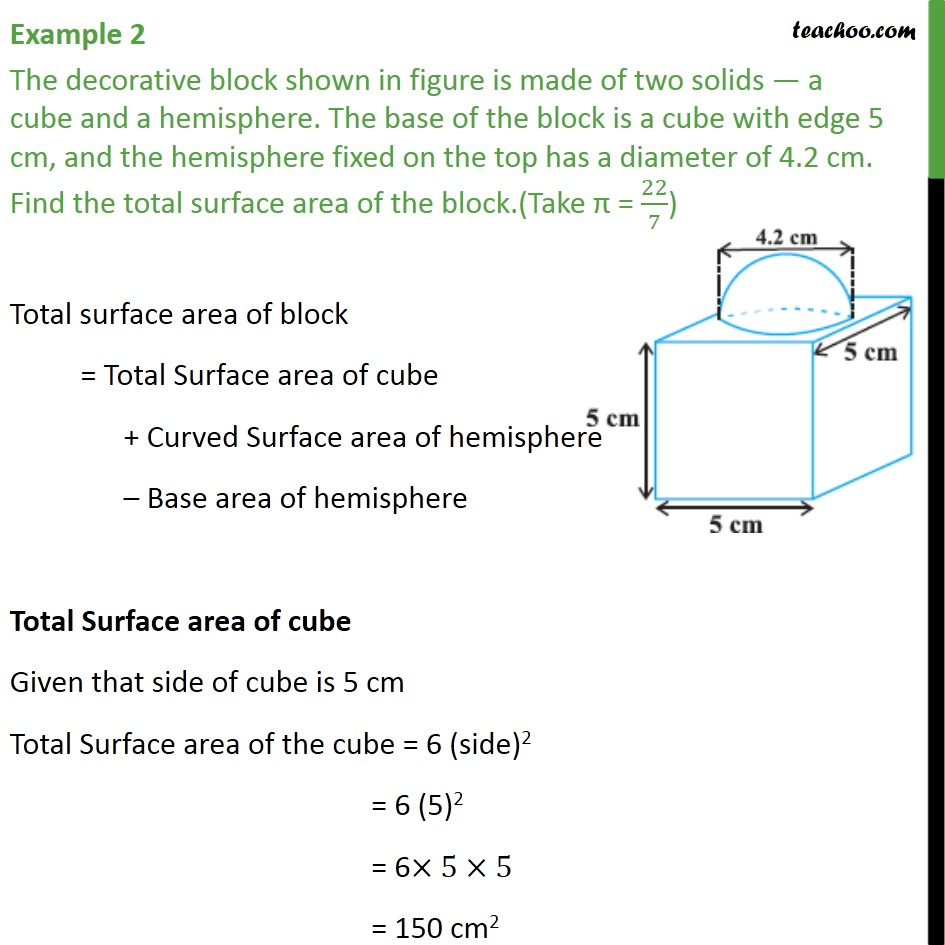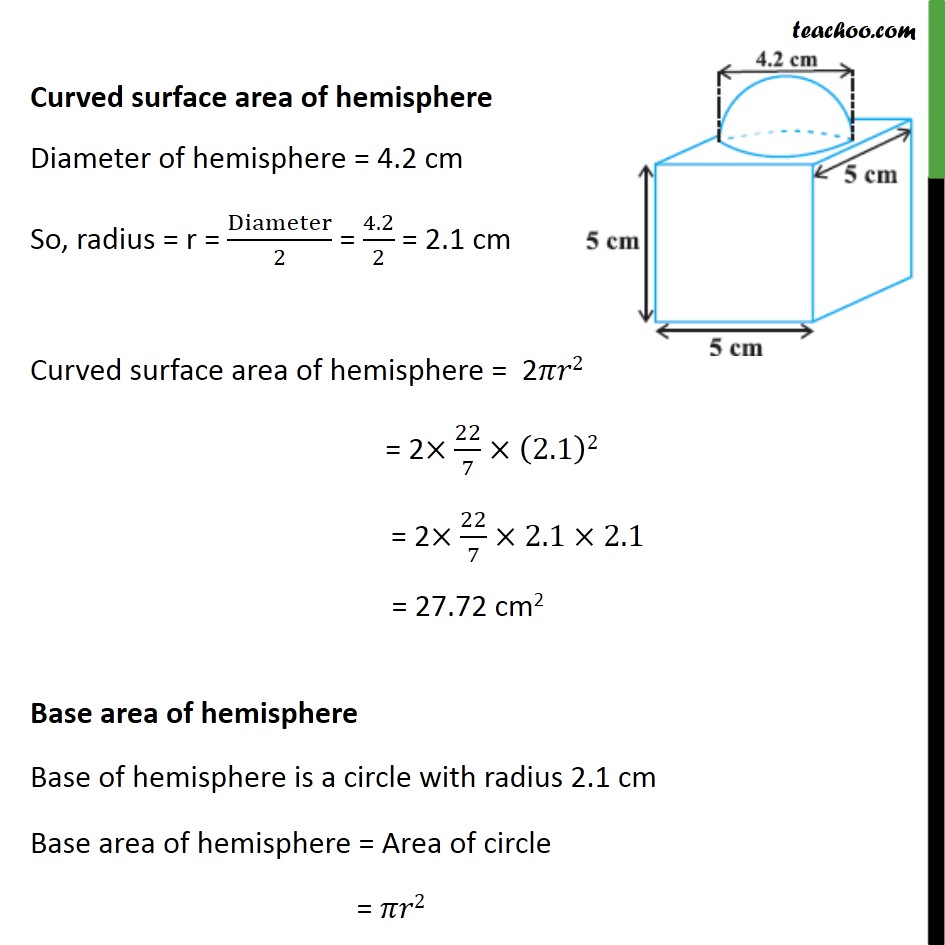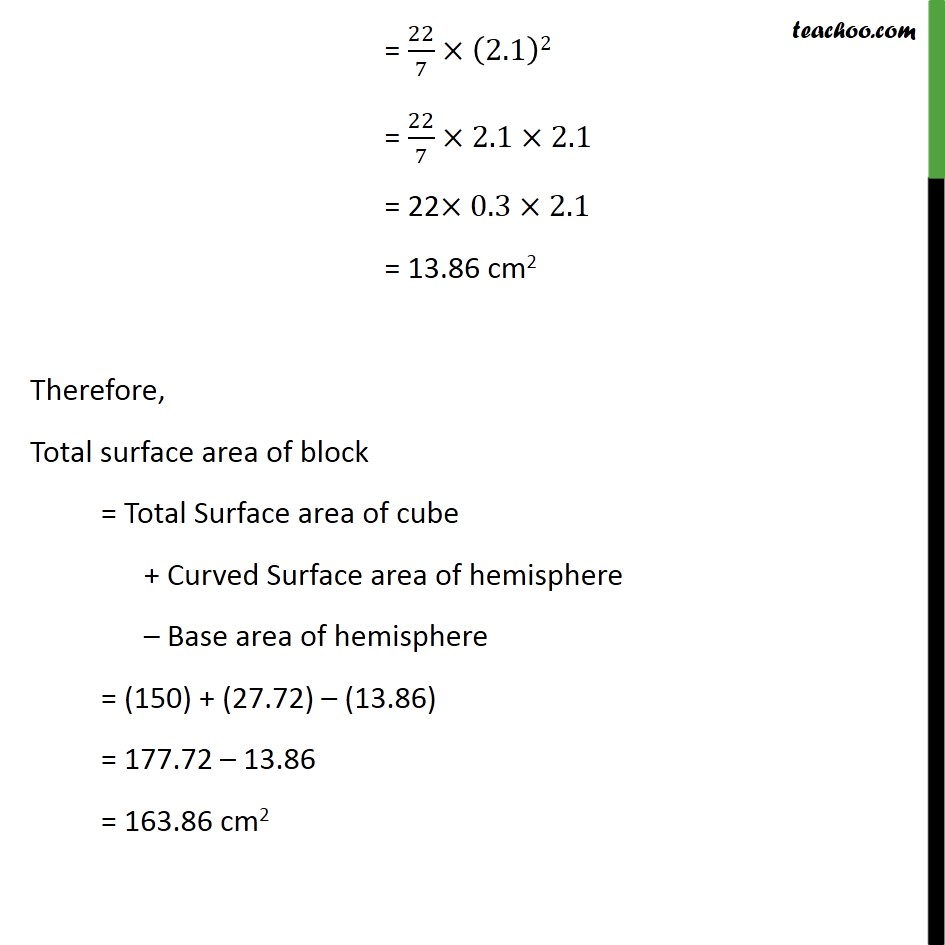1. Chapter 13 Class 10 Surface Areas and Volumes (Term 2)
2. Serial order wise
3. Examples

Transcript

Example 2 The decorative block shown in figure is made of two solids — a cube and a hemisphere. The base of the block is a cube with edge 5 cm, and the hemisphere fixed on the top has a diameter of 4.2 cm. Find the total surface area of the block.(Take π = 22/7) Total surface area of block = Total Surface area of cube + Curved Surface area of hemisphere – Base area of hemisphere Total Surface area of cube Given that side of cube is 5 cm Total Surface area of the cube = 6 (side)2 = 6 (5)2 = 6×5×5 = 150 cm2 Curved surface area of hemisphere Diameter of hemisphere = 4.2 cm So, radius = r = Diameter/2 = 4.2/2 = 2.1 cm Curved surface area of hemisphere = 2𝜋𝑟2 = 2×22/7×(2.1)2 = 2×22/7×2.1×2.1 = 27.72 cm2 Base area of hemisphere Base of hemisphere is a circle with radius 2.1 cm Base area of hemisphere = Area of circle = 𝜋𝑟2 = 22/7×(2.1)2 = 22/7×2.1×2.1 = 22×0.3×2.1 = 13.86 cm2 Therefore, Total surface area of block = Total Surface area of cube + Curved Surface area of hemisphere – Base area of hemisphere = (150) + (27.72) – (13.86) = 177.72 – 13.86 = 163.86 cm2